• 属性动画Rotation如何以中心轴旋转
2021-05-26 11:31:39

//这个是按照某一点进行旋转，默认是view的

ObjectAnimator oaAnimator=ObjectAnimator.ofFloat(imageView1, "rotation", 0,360);

//如果不指定中心点的话就是按照图标自己的中心进行旋转

imageView1.setPivotX(100);//设置指定旋转中心点X坐标

imageView1.setPivotY(100);//设置指定旋转中心点X坐标，注意的是这个点(100,100)是想对于view的坐标，不是屏幕的左上角的0，0位置，有了这你就可以实现和补间动画一样的效果

oaAnimator.setDuration(5000);

oaAnimator.start();

//这个是以Y中心轴进行旋转

ObjectAnimator oaY=ObjectAnimator.ofFloat(imageView1, "rotationY", 0,360);

oaY.setDuration(5000);

oaY.start();

更多相关内容
• 张正友标定以及旋转轴标定，通过输入几张图片，来求得摄像机内外参数以及旋转轴的参数。
• 利用宏程序用来自动计算已知工件上任意点在旋转任意角度后的机械坐标，并自动地填写进坐标寄存器中，这样我们就可以直接把这个点当成原点来编程。
• 给定的程序是生成椭圆并相对于中心旋转它...... 任何人都可以为我提供一个程序来旋转椭圆的焦点......？matlab
• 本文实例讲述了Android编程实现RotateAnimation设置中心旋转动画效果。分享给大家供大家参考，具体如下： 在xml设置： <?xml version=1.0 encoding=utf-8?> <rotate xmlns:android=...
• 通过halcon仿真，基于最小二乘法来求吸嘴或旋转中心，资源包含测试图片，保存的区域，以及相机标定文件，可通过保存内置保存的区域，直接进行边缘检测提取角点，快速完成旋转中心的提取，无需在手动绘制。
• 本方法借助已有的api实现了对任意中心旋转功能，简单易懂易于操作

three.js中自带围绕X,Y,Z轴旋转的api，但要想实现任一方向的中心旋转功能尚未找到简单易懂的资料以供参考。

起初想到的是能否借助四元数来实现？但是四元数还是不太友好，不太容易理解。在吃饭反途中灵光一现，之前看到过有网友借助group实现了中心的自定义，是否同样能借助group来实现旋转轴的自定义呢？

想到这里，顿时激动起来。跑会机房开始验证。

果然可以实现！

原理很简单，借助已有的api即可实现。

首先创建模型，并将其加入一个group中

 let cloud= creatPointCloudByGeometry(geom);

 let group=new THREE.Group();
group.position.set(0,0,0);


通过模型自带的旋转api控制模型围绕自身的哪个轴旋转，即确定相对自身的旋转方向。

cloud.rotation.x += gui.x;
cloud.rotation.y += gui.y;
cloud.rotation.z += gui.z;


通过旋转group实现模型的旋转相对于场景方向的控制

 group.rotation.x=gui.GroupX;
group.rotation.y=gui.GroupY;
group.rotation.z=gui.GroupZ;


这是我的render函数


function render(){

group.rotation.x=gui.GroupX;
group.rotation.y=gui.GroupY;
group.rotation.z=gui.GroupZ;

if(gui.rotation){
cloud.rotation.x += gui.x;
cloud.rotation.y += gui.y;
cloud.rotation.z += gui.z;
}

renderer.render(scene,camera);

requestAnimationFrame(render);

}


通过dat.gui实现参数的调节，效果很不错。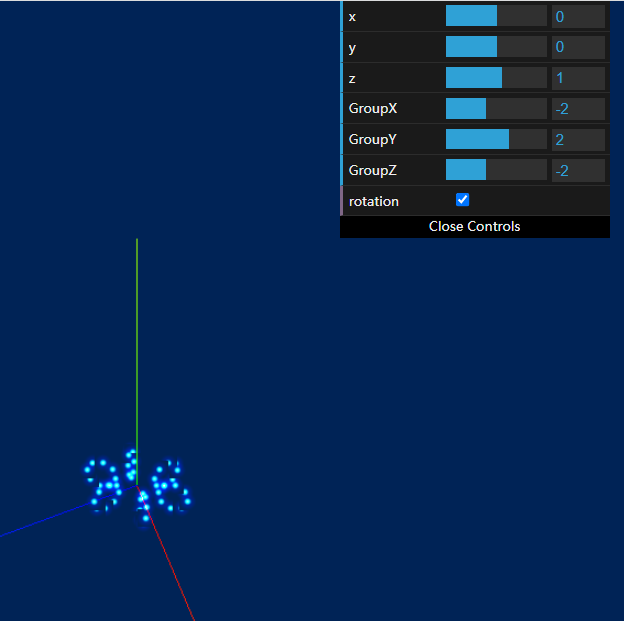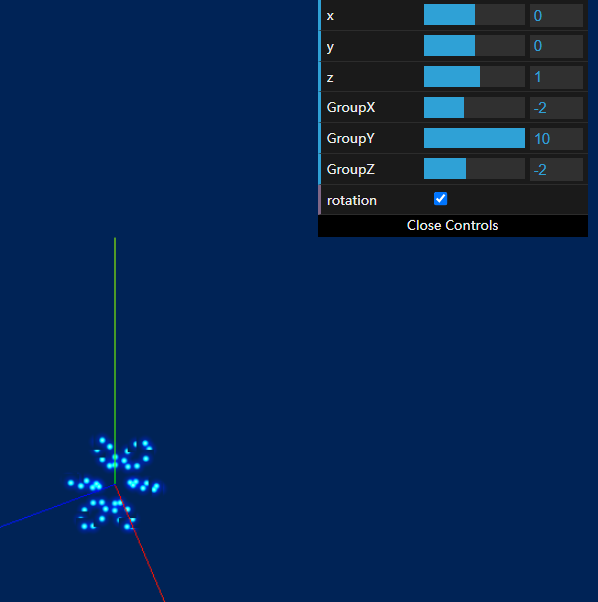总结：本方法借助已有的api实现了对任意轴的中心旋转功能。

思路：

1.通过模型的旋转api确定模型相对自身的旋转方向；

2.通过group的旋转控制模型相对场景的方向

展开全文javascript 前端
• 行业资料-交通装置-一种地下旋转车库及其中心轴.zip
• 主要为大家详细介绍了Unity实现物体沿自身的任意轴向旋转，文中示例代码介绍的非常详细，具有一定的参考价值，感兴趣的小伙伴们可以参考一下
• 基于刚体运动学原理齐次变换矩阵方法建立了包含旋转轴准静态误差和旋转轴中心轴线与三个直线轴间垂直度误差的误差模型；用激光跟踪仪测出旋转轴循圆运动到不同角度位置时其端面上点的空间坐标，由此建立旋转轴误差...
• 　1）采用光纤旋转连接器，但是该器件必须安装于旋转轴中心或允许偏离中心很小的距离，在一些旋转轴中需要进行液压、气压传动以及需要布设其他线路的场合则不适用，且机械加工精度要求也较高； 　2）采用集电环，...
• 文章地址在：https://blog.csdn.net/mazhiyuan1981/article/details/108882385
• 今天小编就为大家分享一篇python实现一个点绕另一个点旋转后的坐标，具有很好的参考价值，希望对大家有所帮助。一起跟随小编过来看看吧
• 问题描述 课题目前是使用DEFORM软件做搅拌摩擦焊接温度场仿真，模型分为搅拌工具（即搅拌头）与焊件，搅拌头在运动过程中有...DEFORM确定旋转轴是通过向量来表示的，所以绕X轴旋转为（1，0，0）；绕Y轴旋转为（0，1，0

# 问题描述

课题目前是使用DEFORM软件做搅拌摩擦焊接温度场仿真，模型分为搅拌工具（即搅拌头）与焊件，搅拌头在运动过程中有时候需要更改不同的倾角完成焊接，DEFORM中控制part运动的方法是自动寻找part中心与旋转中心，因为软件具有一定缺陷，所以有时候自动寻找中心轴会不好使，所以需要自己确定坐标。

# 中心如何确定

软件中Axis-Center自动确定是准确的，而Center上方单元格内的坐标有时候会出问题。
DEFORM确定旋转轴是通过向量来表示的，所以绕X轴旋转为（1，0，0）；绕Y轴旋转为（0，1，0）；绕Z轴旋转为（0，0，1）.
模型如下图所示，搅拌头沿Y轴正半轴进给完成焊接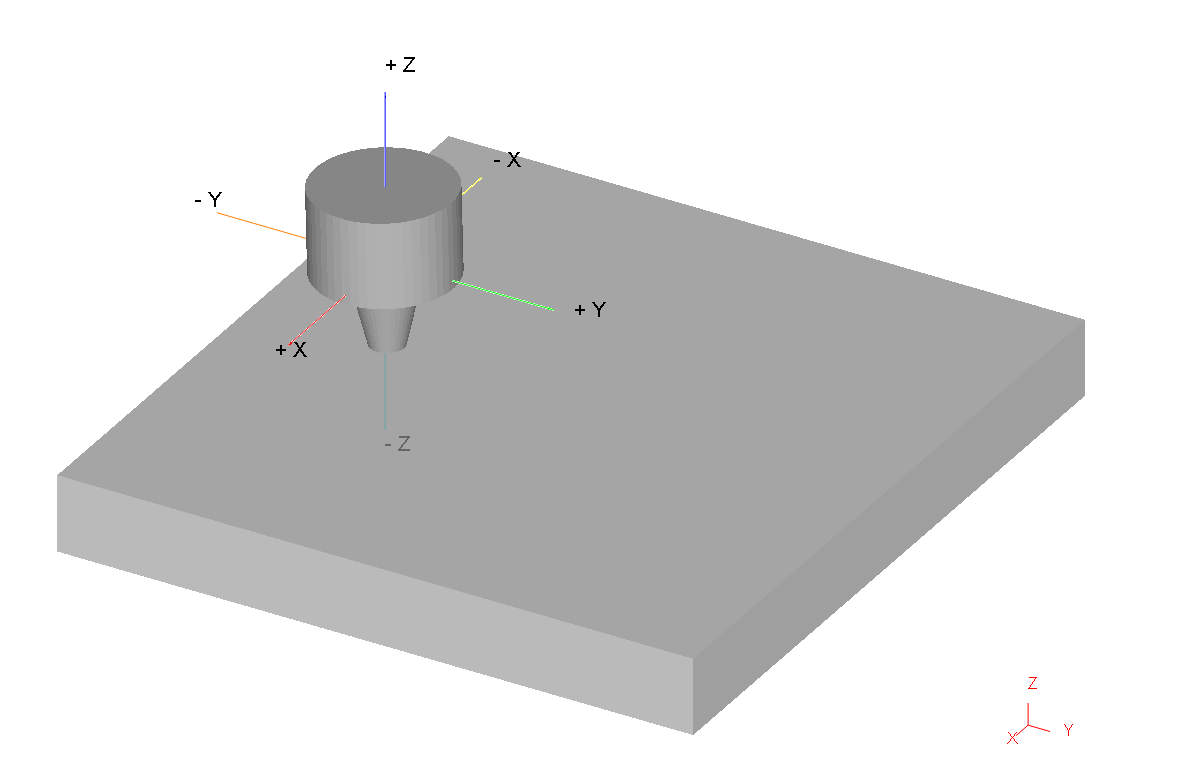搅拌头倾斜一般是绕X轴旋转一定角度，假设旋转2°，如下图所示：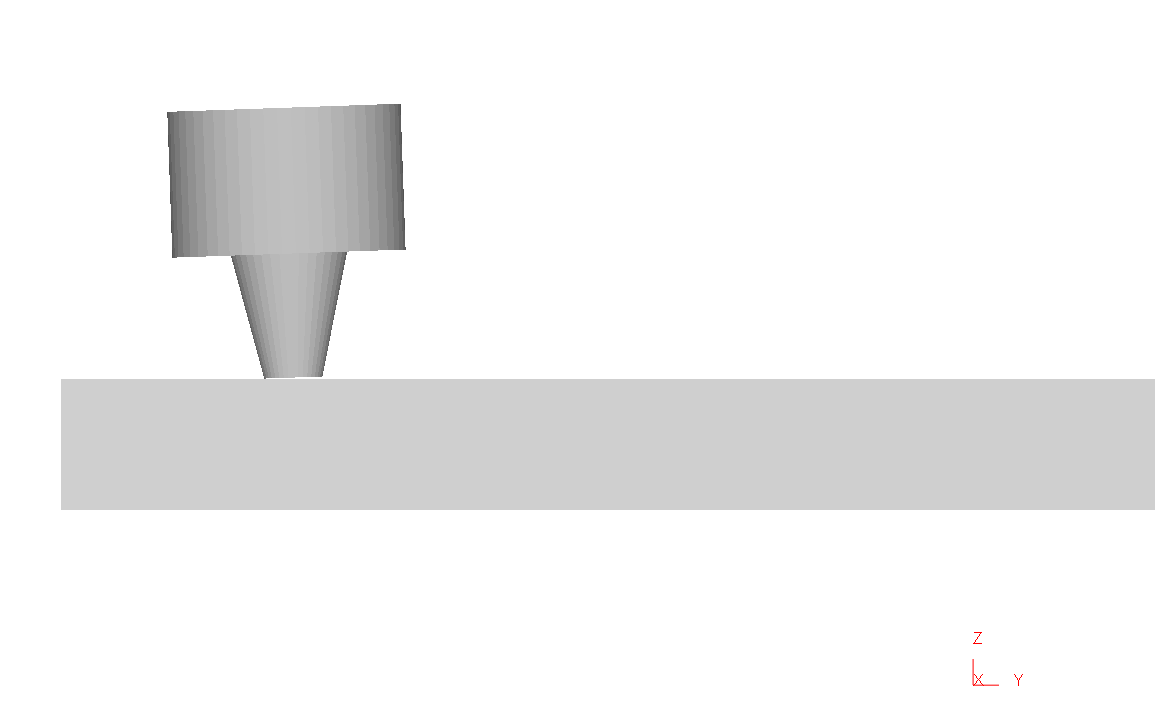此时，输入的向量应该为（0，-sin2°，cos2°），如下图所示，其实相当于向量的分解，如果焊接方向为其他或者是其他工况下也可以通过此方法确定。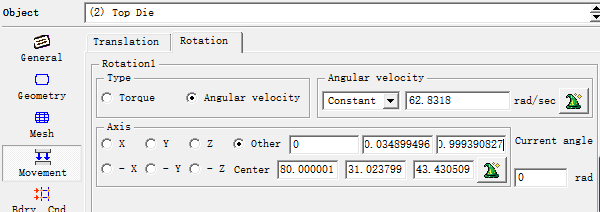负号没有显示出来，记得为-sin2°！

# 因为有同学询问过关于这方面的问题，所以放在这里，需要自取

展开全文• 七年级数学下册第10章对称平移和旋转10.4中心对称作业设计新版华东师大版
• 1 右手坐标系 右手坐标系如下图： X：拇指指向X Y：食指指向Y Z：中指指向Z ...例如X顺时针旋转90度，为了验证这个旋转结果是否正确，我们需要弄清楚这个90度是怎么旋转的： 可以从原点（0,0,0）向X

# 1 右手坐标系

右手坐标系如下图：

• X拇指指向X轴
• Y食指指向Y轴
• Z中指指向Z轴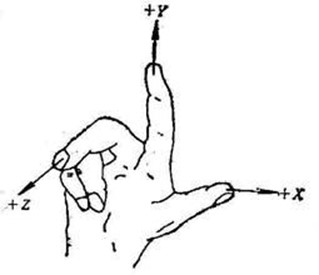可能很多人还是记不住，我个人总结两个小技巧，很容易牢记右手坐标系

• X、Y、Z的顺序刚好也是对应手指大拇指、食指、中指也是顺序排列的
• 做一个右手坐标系的姿势，然后让食指（Y轴）指向别人的姿势，很容易就可以把右手坐标系画出来

如下，是我画的右手坐标系：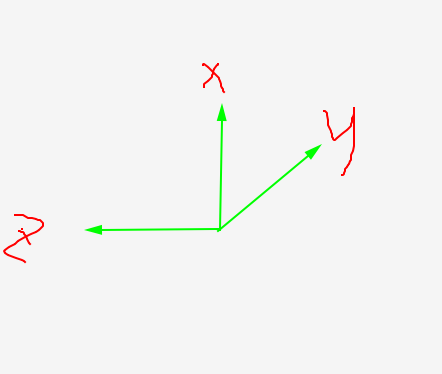## 1.1 旋转90度是什么样的

例如X轴顺时针旋转90度，为了验证这个旋转结果是否正确，我们需要弄清楚这个90度是怎么旋转的：

• 可以从原点（0,0,0）向X轴看，此时就可以把三维坐标系降维到二维坐标系YOZ
• 绕X轴旋转的过程中，自然X轴的坐标是不变的，只有Y和Z是变化的。

例如：(2, 0, 3)绕X轴顺时针旋转90度，旋转后的坐标为：(2, 3, 0)

# 2 XYZ空间内某点绕X、Y、Z轴旋转一次

首先假设：

• 旋转前坐标为： ( x , y , z ) (x, y, z)
• 旋转后坐标为： ( x ′ , y ′ , z ′ ) (x^{\prime}, y^{\prime}, z^{\prime})

下面用一些内容的简单说明：

1、角度弧度的相互转化（关于角度和弧度的更多介绍参考

• 角度弧度 弧 度 = 角 度 × π 180 弧度 = 角度 \times \frac{\pi}{180}
• 弧度角度 角 度 = 弧 度 × 180 π 角度 = 弧度 \times \frac{180}{\pi}

2、python的math模块和numpy模块计算正弦sin和余弦cos输入的参数值都是弧度制

如下，计算sin90度

>>> import numpy as np
>>> import math
>>> np.sin(90*(np.pi/180))
1.0
>>> math.sin(90*(math.pi/180))
1.0
>>>


## 2.1 XYZ空间内某点绕Z轴旋转γ角

1、绕Z轴旋转：Z轴的坐标是不变化的

[ x ′ , y ′ , z ′ , 1 ] = [ x , y , z , 1 ] [ cos ⁡ γ sin ⁡ γ 0 0 − sin ⁡ γ cos ⁡ γ 0 0 0 0 1 0 0 0 0 1 ] \left[x^{\prime}, y^{\prime}, z^{\prime}, 1\right]=[x, y, z, 1]\left[\begin{array}{cccc} \cos \gamma & \sin \gamma & 0 & 0 \\ -\sin \gamma & \cos \gamma & 0 & 0 \\ 0 & 0 & 1 & 0 \\ 0 & 0 & 0 & 1 \end{array}\right]

然后公式可以表示为：

x ′ = cos ⁡ γ ⋅ x − sin ⁡ γ ⋅ y y ′ = sin ⁡ γ ⋅ x + cos ⁡ γ ⋅ y z ′ = z \begin{array}{l} x^{\prime}=\cos \gamma \cdot x-\sin \gamma \cdot y \\ y^{\prime}=\sin \gamma \cdot x+\cos \gamma \cdot y \\ z^{\prime}=z \end{array}

2、python实现XYZ空间内某点绕Z轴旋转γ角

# 1、绕Z周旋转gamma角
def rotate_Z(x, y, z,  gamma):
gamma = gamma * (np.pi / 180)
x_r = np.cos(gamma)*x - np.sin(gamma)*y
y_r = np.sin(gamma)*x + np.cos(gamma)*y
z_r = z
print(f"{(x, y, z)} rotate {gamma*(180/np.pi)} degrees around the Z-axis,result {(x_r, y_r, z_r)}")
return x_r, y_r, z_r


3、C++实现XYZ空间内某点绕Z轴旋转γ角


//将空间点绕Z轴旋转
//输入参数 x y为空间点原始x y坐标
//thetaz为空间点绕Z轴旋转多少度，角度制范围在-180到180
//outx outy为旋转后的结果坐标
void codeRotateByZ(double x, double y, double thetaz, double& outx, double& outy)
{
double x1 = x;//将变量拷贝一次，保证&x == &outx这种情况下也能计算正确
double y1 = y;
double rz = thetaz * CV_PI / 180;
outx = cos(rz) * x1 - sin(rz) * y1;
outy = sin(rz) * x1 + cos(rz) * y1;

}


## 2.2 XYZ空间内某点绕Y轴旋转β角

1、绕Z轴旋转：Z轴的坐标是不变化的

[ x ′ , y ′ , z ′ , 1 ] = [ x , y , z , 1 ] [ cos ⁡ β 0 − sin ⁡ β 0 0 1 0 0 sin ⁡ β 0 cos ⁡ β 0 0 0 0 1 ] \left[x^{\prime}, y^{\prime}, z^{\prime}, 1\right]=[x, y, z, 1]\left[\begin{array}{cccc} \cos \beta & 0 & -\sin \beta & 0 \\ 0 & 1 & 0 & 0 \\ \sin \beta & 0 & \cos \beta & 0 \\ 0 & 0 & 0 & 1 \end{array}\right]

然后公式可以表示为：

x ′ = cos ⁡ β ⋅ x + sin ⁡ β ⋅ z y ′ = y z ′ = − sin ⁡ β ⋅ x + cos ⁡ β ⋅ z \begin{array}{l} x^{\prime}=\cos \beta \cdot x+\sin \beta \cdot z \\ y^{\prime}=y \\ z^{\prime}=-\sin \beta \cdot x+\cos \beta \cdot z \end{array}

2、python实现XYZ空间内某点绕Y轴旋转β角

# 2、绕Y轴旋转beta角
def rotate_Y(x, y, z, beta):
beta = beta * (np.pi / 180)
x_r = np.cos(beta)*x + np.sin(beta)*z
y_r = y
z_r = -np.sin(beta)*x + np.cos(beta)*z
print(f"{(x, y, z)} rotate {beta*(180/np.pi)} degrees around the Y-axis,result {(x_r, y_r, z_r)}")
return x_r, y_r, z_r


3、C++实现XYZ空间内某点绕Y轴旋转β角


//将空间点绕Y轴旋转
//输入参数 x z为空间点原始x z坐标
//thetay为空间点绕Y轴旋转多少度，角度制范围在-180到180
//outx outz为旋转后的结果坐标
void codeRotateByY(double x, double z, double thetay, double& outx, double& outz)
{
double x1 = x;
double z1 = z;
double ry = thetay * CV_PI / 180;
outx = cos(ry) * x1 + sin(ry) * z1;
outz = cos(ry) * z1 - sin(ry) * x1;
}


## 2.3 XYZ空间内某点绕X轴旋转α角

1、绕Z轴旋转：Z轴的坐标是不变化的

[ x ′ , y ′ , z ′ , 1 ] = [ x , y , z , 1 ] [ 1 0 0 0 0 cos ⁡ α sin ⁡ α 0 0 − sin ⁡ α cos ⁡ α 0 0 0 0 1 ] \left[x^{\prime}, y^{\prime}, z^{\prime}, 1\right]=[x, y, z, 1]\left[\begin{array}{cccc} 1 & 0 & 0 & 0 \\ 0 & \cos \alpha & \sin \alpha & 0 \\ 0 & -\sin \alpha & \cos \alpha & 0 \\ 0 & 0 & 0 & 1 \end{array}\right]

然后公式可以表示为：

x ′ = x y ′ = cos ⁡ α ⋅ y − sin ⁡ α ⋅ z z ′ = sin ⁡ α ⋅ y + cos ⁡ α ⋅ z \begin{array}{l} x^{\prime}=x \\ y^{\prime}=\cos \alpha \cdot y-\sin \alpha \cdot z \\ z^{\prime}=\sin \alpha \cdot y+\cos \alpha \cdot z \end{array}

2、python实现XYZ空间内某点绕X轴旋转α角

# 3、绕X轴旋转alpha角
def rotate_X(x, y, z, alpha):
alpha = alpha * (np.pi / 180)
x_r = x
y_r = np.cos(alpha)*y - np.sin(alpha)*z
z_r = np.sin(alpha)*y + np.cos(alpha)*z
print(f"{(x, y, z)} rotate {alpha*(180/np.pi)} degrees around the X-axis,result {(x_r, y_r, z_r)}")
return x_r, y_r, z_r


3、C++实现XYZ空间内某点绕X轴旋转α角


//将空间点绕X轴旋转
//输入参数 y z为空间点原始y z坐标
//thetax为空间点绕X轴旋转多少度，角度制范围在-180到180
//outy outz为旋转后的结果坐标
void codeRotateByX(double y, double z, double thetax, double& outy, double& outz)
{
double y1 = y;//将变量拷贝一次，保证&y == &y这种情况下也能计算正确
double z1 = z;
double rx = thetax * CV_PI / 180;
outy = cos(rx) * y1 - sin(rx) * z1;
outz = cos(rx) * z1 + sin(rx) * y1;


完整代码：

import numpy as np

# 1、绕Z周旋转gamma角

def rotate_Z(x, y, z,  gamma):
gamma = gamma * (np.pi / 180)
x_r = np.cos(gamma)*x - np.sin(gamma)*y
y_r = np.sin(gamma)*x + np.cos(gamma)*y
z_r = z
print(f"{(x, y, z)} rotate {gamma*(180/np.pi)} degrees around the Z-axis,result {(x_r, y_r, z_r)}")
return x_r, y_r, z_r

# 2、绕Y轴旋转beta角
def rotate_Y(x, y, z, beta):
beta = beta * (np.pi / 180)
x_r = np.cos(beta)*x + np.sin(beta)*z
y_r = y
z_r = -np.sin(beta)*x + np.cos(beta)*z
print(f"{(x, y, z)} rotate {beta*(180/np.pi)} degrees around the Y-axis,result {(x_r, y_r, z_r)}")
return x_r, y_r, z_r

# 3、绕X轴旋转alpha角
def rotate_X(x, y, z, alpha):
alpha = alpha * (np.pi / 180)
x_r = x
y_r = np.cos(alpha)*y - np.sin(alpha)*z
z_r = np.sin(alpha)*y + np.cos(alpha)*z
print(f"{(x, y, z)} rotate {alpha*(180/np.pi)} degrees around the X-axis,result {(x_r, y_r, z_r)}")
return x_r, y_r, z_r

# https://zh.wikipedia.org/wiki/%E6%97%8B%E8%BD%AC%E7%9F%A9%E9%98%B5
# 可能是坐标系的不同，维基百科上的斜对角的sin的负号，和我们使用的刚好是交换过来的

if __name__ == '__main__':
# 1、绕Z轴旋转，Z值不变
# 点(2, 0, 12)绕Z轴旋转90度，旋转后 (0, 2, 12)
rotate_Z(2, 0, 12, 90)

# 2、绕Y轴旋转，Y值不变
# 点(3, 4, 0)绕Y轴顺时针旋转90度，旋转后 (0, 4, -3)
rotate_Y(3, 4, 0, 90)

# 3、绕X轴旋转，X值不变
# 点(5, 0, 7)绕X轴顺时针旋转90度，旋转后 (5, -7, 0)
rotate_X(5, 0, 7, 90)

x, y, z = 1, 2, 3
x, y, z = rotate_X(x, y, z, 90)
x, y, z = rotate_Y(x, y, z, -90)
x, y, z = rotate_Z(x, y, z, -90)
# 结果：(1,2,3) -> (-3, 2, 1)

x, y, z = 1, 2, 3
x, y, z = rotate_Z(x, y, z, 90)
x, y, z = rotate_Y(x, y, z, -90)
x, y, z = rotate_X(x, y, z, -90)
# 结果： (1,2,3) - > (-3, -2, -1)
# 因此不同的旋转顺序会导致旋转的结果不一样



如果你看维基百科，会发现在维基百科上我们绕每个轴的旋转矩阵，斜对角的两个sin正弦值的正负号刚好是交换过来的，这个可能就可坐标系的选择有关了，我们这里选择的是右手坐标系

旋转变换参考
参考：https://www.cnblogs.com/singlex/p/3DPointRotate.html

展开全文• 怎样可以设置3D旋转的中心轴啊 比如我一张图片要以图片自己的中心为旋转点，以一条与X轴成320度，与Y轴成-25度的中心轴旋转，就是做出类似3D星云旋转画面的效果，要怎么设置啊3d web前端 图片
• 电子政务-五联动加工中心的电主轴旋转接头冷却装置.zip
• 需求是要实现给图片添加任意角度旋转的文字，文字的旋转中心要是在文字区域中央，就像 CSS 的 rotate 函数一样的效果。如下：   分析&思路 Graphics 类有个 RotateTransform 方法，可以传入任意角度的值来旋转画板...c# matrix
• 可以修改旋转速度、半径、旋转中心位置，旋转平面的角度
• 并且矩阵的，即为变化后的值，，即为变化后的值，，即为变化后的值。 仿射变换的公式为： 同样的，我们可以通过引入齐次坐标来表示仿射变换，公式为： 例如缩放s倍的矩阵为： 旋转变换 相比...
• 九点标定：将像素坐标转为物理世界坐标 走九宫格，间隔适中 旋转中心标定：获得旋转中心 旋转6个点，间隔5-7°
• Halcon|通过旋转前后3维坐标点求旋转轴及旋转角度绕空间任意轴的旋转矩阵Halcon求解旋转矩阵求旋转轴矢量及旋转角度求旋转轴上一点小结 绕空间任意轴的旋转矩阵 绕空间任意轴旋转的旋转矩阵为： 点击查看图片来源及...Halcon 计算机视觉
• ## 机器视觉——旋转中心的标定

千次阅读 多人点赞 2021-05-20 17:17:11
旋转中心的计算使用 1. 为什么要标定旋转中心 2. 如何标定旋转中心 2.1 标定旋转中心的操作步骤 2.2 三点拟合圆心代码： 3. halcon多点拟合圆的方法 4. 如何使用旋转中心计算偏移量 5. 计算一点绕另一点旋转一定...C# halcon
• 该 demo 演示了行星自转（绕任意轴旋转）、行星公转（绕任意点旋转）、镜头拉近/复位（平滑旋转）、行星环（自定义环形体）、行星轴（自定义胶囊体） 02 实现方法 1自定义环形体/胶囊体 编辑器提供的环...
• 用css实现图片斜侧着旋转效果 一、原始图 一、最终效果图 底部图片例子： 一、html代码 <div class="active-box" v-show="index == activeIndex"> <img src="../assets/imgs/home/photosphere.png" ...css3...